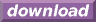#Calcute: freeware calculator

## Hyperbolic Cotangent (coth)

Calcute implements the hyperbolic cotangent function with the coth function.

```coth(1)
0.761594155956
```

It is defined as the inverse of the hyperbolic tangent function coth(x) = 1 / tanh(x) or equivalently coth(x) = cosh(x) / sinh(x). Note that this definition refers to a multiplicative inverse; the hyperbolic cotangent is not the same as the inverse hyperbolic tangent (atanh) function.